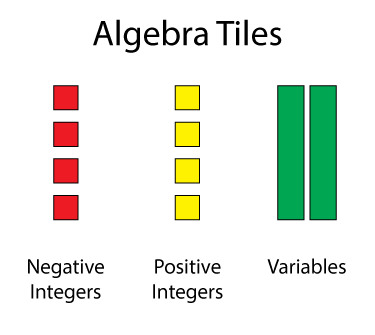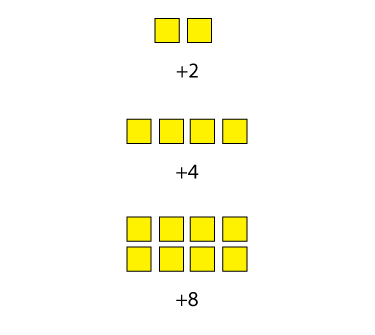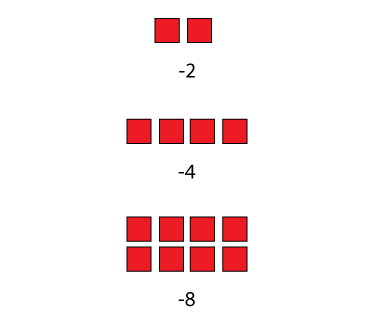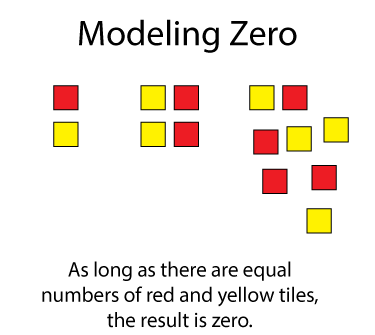# Video Transcript: Algebra Tiles: Modeling Positive Integers Using Algebra Tiles

## What Are Algebra Tiles?

### Numerical Models

Algebra Tiles are a very useful numerical model for positive integers, negative integers, and even variables.Here are examples of modeling positive integers using algebra tiles.Here are examples of modeling negative integers using algebra tiles.You can even model zero by placing a positive tile and a negative tile together. This is called a zero pair. There are multiple ways of modeling zero, so long as equal numbers of red and yellow tiles are used.### Modeling Expressions

Algebra tiles can also be used to model algebraic expressions. To model such expressions, use the tall green tile to model the variable.### Modeling Equations

You can also use algebra tiles to model and solve equations.Use zero pairs to solve for the variable.This is the transcript that goes with the video segment entitled Video: Algebra Tiles: Modeling Positive Integers Using Algebra Tiles.